## The DFT “à Pied”: Mastering The Fourier Transform in One Day

Posted by neuronaut on September 21, 1999
Tutorials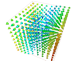If you’re into signal processing, you will no doubt say that the headline is a very tall claim. I would second this. Of course you can’t learn all the bells and whistles of the Fourier transform in one day without practising and repeating and eventually delving into the maths. However, this online course will provide you with the basic knowledge of how the Fourier transform works, why it works and why it can be very simple to comprehend when we’re using a somewhat unconventional approach. The important part: you will learn the basics of the Fourier transform completely without any maths that goes beyond adding and multiplying numbers! I will try to explain the Fourier transform in its practical application to audio signal processing in no more than six paragraphs below.

Step 1: Some simple prerequisites
What you need to understand the following paragraphs are essentially four things: how to add numbers, how to multiply and divide them and what a sine, a cosine and a sinusoid is and how they look. Obviously, I will skip the first two things and just explain a bit the last one. You probably remember from your days at school the ‘trigonometric functions’* that were somehow mysteriously used in conjunction with triangles to calculate the length of its sides from its inner angles and vice versa. We don’t need all these things here, we just need to know how the two most important trigonometric functions, the “sine” and “cosine” look like. This is quite simple: they look like very simple waves with peaks and valleys in them that stretch out to infinity to the left and the right of the observer.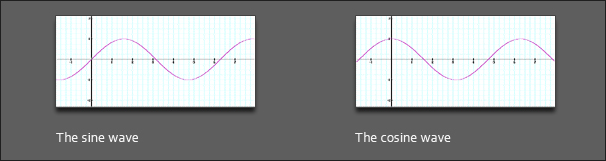As you can see, both waves are periodic, which means that after a certain time, the period, they look the same again. Also, both waves look alike, but the cosine wave appears to start at its maximum, while the sine wave starts at zero. Now in practice, how can we tell whether a wave we observe at a given time started out at its maximum, or at zero? Good question: we can’t. There’s no way to discern a sine wave and a cosine wave in practice, thus we call any wave that looks like a sine or cosine wave a “sinusoid”, which is Greek and translates to “sinus-like”. An important property of sinusoids is “frequency”, which tells us how many peaks and valleys we can count in a given period of time. High frequency means many peaks and valleys, low frequency means few peaks and valleys: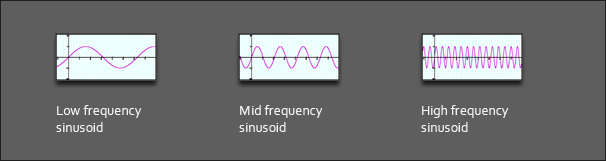Step 2: Understanding the Fourier Theorem
Jean-Baptiste Joseph Fourier was one of those children parents are either proud or ashamed of, as he started throwing highly complicated mathematical terms at them at the age of fourteen. Although he did a lot of important work during his lifetime, the probably most significant thing he discovered had to do with the conduction of heat in materials. He came up with an equation that described how the heat would travel in a certain medium, and solved this equation with an infinite series of trigonometric functions (the sines and cosines we have discussed above). Basically, and related to our topic, what Fourier discovered boils down to the general rule that every signal, however complex, can be represented by a sum of sinusoid functions that are individually mixed.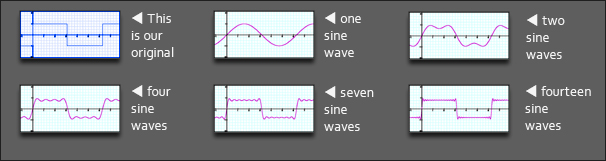What you see here is our original signal, and how it can be approximated by a mixture of sines (we will call them partials) that are mixed together in a certain relationship (a ‘recipe’). We will talk about that recipe shortly. As you can see, the more sines we use the more accurately does the result resemble our original waveform. In the ‘real’ world, where signals are continuous, ie. you can measure them in infinitely small intervals at an accuracy that is only limited by your measurement equipment, you would need infinitely many sines to perfectly build any given signal. Fortunately, as DSPers we’re not living in such a world. Rather, we are dealing with samples of such ‘realworld’ signals that are measured at regular intervals and only with finite precision. Thus, we don’t need infinitely many sines, we just need a lot. We will also talk about that ‘how much is a lot’ later on. For the moment, it is important that you can imagine that every signal you have on your computer can be put together from simple sine waves, after some cooking recipe.

Step 3: How much is “a lot”
As we have seen, complex shaped waveforms can be built from a mixture of sine waves. We might ask how many of them are needed to build any given signal on our computer. Well, of course, this may be as few as one single sine wave, provided we know how the signal we are dealing with is made up. In most cases, we are dealing with realworld signals that might have a very complex structure, so we do not know in advance how many ‘partial’ waves there are actually present. In this case, it is very reassuring to know that if we don’t know how many sine waves constitute the original signal there is an upper limit to how many we will need. Still, this leaves us with the question of how many there actually are. Let’s try to approach this intuitively: assume we have 1000 samples of a signal. The sine wave with the shortest period (ie. the most peaks and valleys in it) that can be present has alternating peaks and valleys for every sample. So, the sine wave with the highest frequency has 500 peaks and 500 valleys in our 1000 samples, with every other sample being a peak. The black dots in the following diagram denote our samples, so the sine wave with the highest frequency looks like this:Now let’s look how low the lowest frequency sine wave can be. If we are given only one single sample point, how would we be able to measure peaks and valleys of a sine wave that goes through this point? We can’t, as there are many sine waves of different periods that go through this point.So, a single data point is not enough to tell us anything about frequency. Now, if we were given two samples, what would be the lowest frequency sine wave that goes through these two points? In this case, it is much simpler. There is one very low frequency sine wave that goes through the two points. It looks like this:Imagine the two leftmost points being two nails with a string spanned between them (the diagram depicts three data points as the sine wave is periodic, but we really only need the leftmost two to tell its frequency). The lowest frequency we can see is the string swinging back and forth between the two nails, like our sine wave does in the diagram between the two points to the left. If we have 1000 samples, the two ‘nails’ would be the first and the last sample, ie. sample number 1 and sample number 1000. We know from our experience with musical instruments that the frequency of a string goes down when its length increases. So we would expect that our lowest sine wave gets lower in frequency when we move our nails farther away from each other. If we choose 2000 samples, for instance, the lowest sine wave will be much lower since our ‘nails’ are now sample number 1 and sample number 2000. In fact, it will be twice as low, since our nails are now twice as far away as in the 1000 samples. Thus, if we have more samples, we can discern sine waves of a lower frequency since their zero crossings (our ‘nails’) will move farther away. This is very important to understand for the following explanations.

As we can also see, after two ‘nails’ our wave starts to repeat with the ascending slope (the first and the third nail are identical). This means that any two adjacent nails embrace exactly one half of the complete sine wave, or in other words either one peak or one valley, or 1/2 period.

Summarizing what we have just learned, we see that the upper frequency of a sampled sine wave is every other sample being a peak and a valley and the lower frequency bound is half a period of the sine wave which is just fitting in the number of samples we are looking at. But wait – wouldn’t this mean that while the upper frequency remains fixed, the lowest frequency would drop when we have more samples? Exactly! The result of this is that we will need more sine waves when we want to put together longer signals of unknown content, since we start out at a lower frequency.

All well and good, but still we don’t know how many of these sine waves we finally need. As we now know the lower and upper frequency any partial sine wave can have, we can calculate how many of them fit in between these two limits. Since we have nailed our lowest partial sine wave down to the leftmost and rightmost samples, we require that all other sine waves use these nails as well (why should we treat them differently? All sine waves are created equal!). Just imagine the sine waves were strings on a guitar attached to two fixed points. They can only swing between the two nails (unless they break), just like our sine waves below. This leads to the relationship that our lowest partial (1) fits in with 1/2 period, the second partial (2) fits in with 1 period, the third partial (3) fits in with 1 1/2 period asf. into the 1000 samples we are looking at.

Graphically, this looks like this: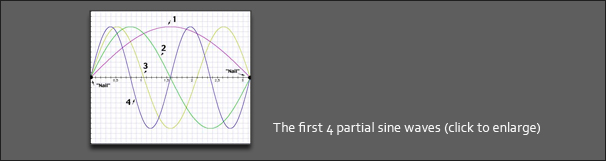Now if we count how many sine waves fit in our 1000 samples that way, we will find that we need exactly 1000 sine waves added together to represent the 1000 samples. In fact, we will always find that we need as many sine waves as we had samples.

In the previous paragraph we have seen that any given signal on a computer can be built from a mixture of sine waves. We have considered their frequency and what frequency the lowest and highest sine waves need to have to perfectly reconstruct any signal we analyze. We have seen that the number of samples we are looking at is important for determining the lowest partial sine wave that is needed, but we have not yet discussed how the actual sine waves have to be mixed to yield a certain result. To make up any given signal by adding sine waves, we need to measure one additional aspect of them. As a matter of fact, frequency is not the only thing we need to know. We also need to know the amplitude of the sine waves, ie. how much of each sine wave we need to mix together to reproduce our input signal. The amplitude is the height of the peaks of a sine wave, ie. the distance between the peak and our zero line. The higher the amplitude, the louder it will sound when we listen to it. So, if you have a signal that has lots of bass in it you will no doubt expect that there must be a greater portion of lower frequency sine waves in the mix than there are higher frequency sine waves. So, generally, the low frequency sine waves in a bassy sound will have a higher amplitude than the high frequency sine waves. In our analysis, we will need to determine the amplitude of each partial sine wave to complete our recipe.

Step 5: About apples and oranges
If you are still with me, we have almost completed our journey towards the Fourier transform. We have learned how many sine waves we need, that this number depends on the number of samples we are looking at, that there is a lower and upper frequency boundary and that we somehow need to determine the amplitude of the individual partial waves to complete our recipe. We’re still not clear, however, on how we can determine the actual recipe from our samples. Intuitively, we would say that we could find the amplitudes of the sine waves somehow by comparing a sine wave of known frequency to the samples we have measured and find out how ‘equal’ they are. If they are exactly equal, we know that the sine wave must be present at the same amplitude, if we find our signal to not match our reference sine wave at all we would expect this frequency not to be present. Still, how could we effectively compare a known sine wave with our sampled signal? Fortunately, DSPers have already figured out how to do this for you. In fact, this is as easy as multipling and adding numbers – we take the ‘reference’ sine wave of known frequency and unit amplitude (this just means that it has an amplitude of 1, which is exactly what we get back from the sin() function on our pocket calculator or our computer) and multiply it with our signal samples. After adding the result of the multiplication together, we will obtain the amplitude of the partial sine wave at the frequency we are looking at.

To illustrate this, here’s a simple C code fragment that does this: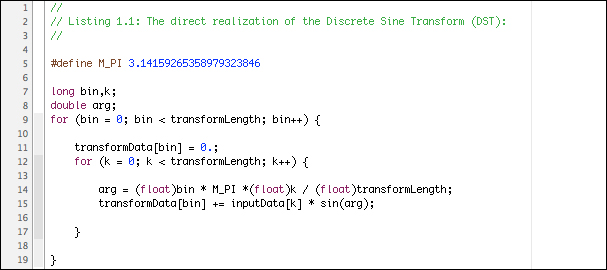This code segment transforms our measured sample points that are stored in inputData[0…transformLength-1] into an array of amplitudes of its partial sine waves transformData[0…transformLength-1]. According to common terminology, we call the frequency steps of our reference sine wave bins, which means that they can be thought of as being ‘containers’ in which we put the amplitude of any of the partial waves we evaluate. The Discrete Sine Transform (DST) is a generic procedure that assumes we have no idea what our signal looks like, otherwise we could use a more efficient method for determining the amplitudes of the partial sine waves (if we, for example, know beforehand that our signal is a single sine wave of known frequency we could directly check for its amplitude without calculating the whole range of sine waves. An efficient approach for doing this based on the Fourier theory can be found in the literature under the name the “Goertzel” algorithm).

For those of you who insist on an explanation for why we calculate the sine transform that way: As a very intuitive approach to why we multiply with a sine wave of known frequency, imagine that this corresponds roughly to what in the physical world happens when a ‘resonance’ at a given frequency takes place in a system. The sin(arg) term is essentially a resonator that gets excited by our input waveform. If the input has a partial at the frequency we’re looking at, its output will be the amplitude of the resonance with the reference sine wave. Since our reference wave is of unit amplitude, the output is a direct measure of the actual amplitude of the partial at that frequency. Since a resonator is nothing but a simple filter, the transform can (admittedly under somewhat relaxed conditions) be seen as a having the features of a bank of very narrow band pass filters that are centered around the frequencies we’re evaluating. This helps explaining the fact why the Fourier transform provides an efficient tool for performing filtering of signals.

Just for the sake of completeness: of course, the above routine is invertible, our signal can (within the limits of our numerical precision) be perfectly reconstructed when we know its partial sine waves, by simply adding sine waves together. This is left as an exercise to the reader. The same routine can be changed to work with cosine waves as basis functions – we simply need to change the sin(arg) term to cos(arg) to obtain the direct realization of the Discrete Cosine Transform (DCT).

Now, as we have discussed in the very first paragraph of this article, in practice we have no way to classify a measured sinus-like function as sine wave or cosine wave. Instead we are always measuring sinusoids, so both the sine and cosine transform are of no great use when we are applying them in practice, except for some special cases (like image compression where each image might have features that are well modelled by a cosine or sine basis function, such as large areas of the same color that are well represented by the cosine basis function). A sinusoid is a bit more general than the sine or cosine wave in that it can start at an arbitrary position in its period. We remember that the sine wave always starts out at zero, while the cosine wave starts out at one. When we take the sine wave as reference, the cosine wave starts out 1/4th later in its period. It is common to measure this offset in degree or radians, which are two units commonly used in conjunction with trigonometric functions. One complete period equals 360° (pron. “degree”) or 2π radian (pron. “two pi” with “pi” pronounced like the word “pie”. π is a Greek symbol for the number 3.14159265358979323846… which has some significance in trigonometry). The cosine wave thus has an offset of 90° or π/2. This offset is called the phase of a sinusoid, so looking at our cosine wave we see that it is a sinusoid with a phase offset of 90° or π/2 relative to the sine wave.

So what’s this phase business all about. As we can’t restrict our signal to start out at zero phase or 90° phase all the time (since we are just observing a signal which might be beyond our control) it is of interest to determine its frequency, amplitude and phase to uniquely describe it at any one time instant. With the sine or cosine transform, we’re restricted to zero phase or 90° phase and any sinusoid that has an arbitrary phase will cause adjacent frequencies to show spurious peaks (since they try to ‘help’ the analysis to force-fit the measured signal to a sum of zero or 90° phase functions). It’s a bit like trying to fit a round stone into a square hole: you need smaller round stones to fill out the remaining space, and even more even smaller stones to fill out the space that is still left empty, and so on. So what we need is a transform that is general in that it can deal with signals that are built of sinusoids of arbitrary phase.

Step 6: The Discrete Fourier transform.
The step from the sine transform to the Fourier transform is simple, making it in a way more ‘general’. While we have been using a sine wave for each frequency we measure in the sine transform, we use both a sine and a cosine wave in the Fourier transform. That is, for any frequency we are looking at we ‘compare’ (or ‘resonate’) our measured signal with both a cosine and a sine wave of the same frequency. If our signal looks much like a sine wave, the sine portion of our transform will have a large amplitude. If it looks like a cosine wave, the cosine part of our transform will have a large amplitude. If it looks like the opposite of a sine wave, that is, it starts out at zero but drops to -1 instead of going up to 1, its sine portion will have a large negative amplitude. It can be shown that the + and – sign together with the sine and cosine phase can represent any sinusoid at the given frequency**.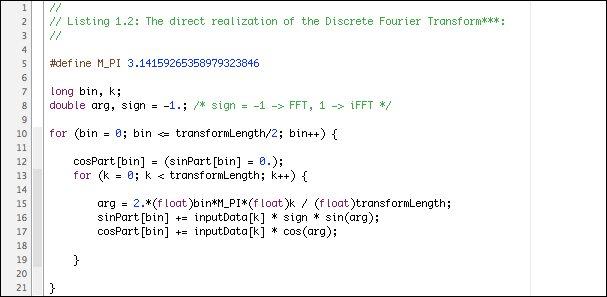We’re still left with the problem of how to get something useful out of the Fourier Transform. I have claimed that the benefit of the Fourier transform over the Sine and Cosine transform is that we are working with sinusoids. However, we don’t see any sinusoids yet, there are still only sines and cosines. Well, this requires an additional processing step: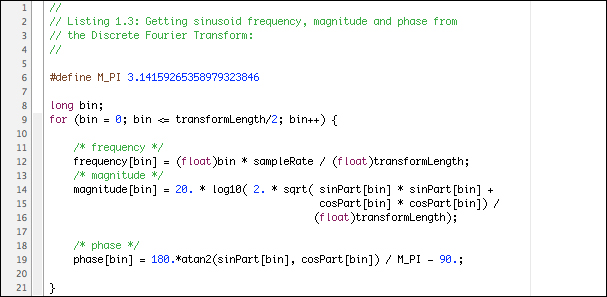After running the code fragment shown in Listing 1.3 on our DFT output, we end up with a representation of the input signal as a sum of sinusoid waves. The k-th sinusoid is described by frequency[k], magnitude[k] and phase[k]. Units are Hz (Hertz, periods per seconds), dB (Decibel) and ° (Degree). Please note that after the post-processing of Listing 1.3 that converts the sine and cosine parts into a single sinusoid, we name the amplitude of the k-th sinusoid the DFT bin “magnitude“, as it will now always be a positive value. We could say that an amplitude of -1.0 corresponds to a magnitude of 1.0 and a phase of either + or -180°. In the literature, the array magnitude[] is called the Magnitude Spectrum of the measured signal, the array phase[] is called the Phase Spectrum of the measured signal at the time where we take the Fourier transform.

As a reference for measuring the bin magnitude in decibels, our input wave is expected to have sample values in the range [-1.0, 1.0), which corresponds to a magnitude of 0dB digital full scale (DFS). As an interesting application of the DFT, listing 1.3 can, for example, be used to write a spectrum analyzer based on the Discrete Fourier Transform.

Conclusion
As we have seen, the Fourier transform and its ‘relatives’, the discrete sine and cosine transform provide handy tools to decompose a signal into a bunch of partial waves. These are either sine or cosine waves, or sinusoids (described by a combination of sine and cosine waves). The advantage of using both the sine and cosine wave simultaneously in the Fourier transform is that we are thus able to introduce the concept of phase which makes the transform more general in that we can use it to efficiently and clearly analyze sinusoids that are neither a pure sine or cosine wave, and of course other signals as well.

The Fourier transform is independent of the signal under examination in that it requires the same number of operations no matter if the signal we are analyzing is one single sinusoid or something else more complicated. This is the reason why the Discrete Fourier transform is called a nonparametric transform, meaning that it is not directly helpful when an ‘intelligent’ analysis of a signal is needed (in the case where we are examining a signal that we know is a sinusoid, we would prefer just getting information about its phase, frequency and magnitude instead of a bunch of sine and cosine waves at some predefined frequencies).

We now also know that we are evaluating our input signal at a fixed frequency grid (our bins) which may have nothing to do with the actual frequencies present in our input signal. Since we choose our reference sine and cosine waves (almost) according to taste with regard to their frequency, the grid we impose on our analysis is artificial. Having said this, it is immediately clear that one will easily encounter a scenario where the measured signal’s frequencies may come to lie between the frequencies of our transform bins. Consequently, a sinusoid that has a frequency that happens to lie between two frequency ‘bins’ will not be well represented in our transform. Adjacent bins that surround the bin closest in frequency to our input wave will try to ‘correct’ the deviation in frequency and thus the energy of the input wave will be smeared over several neighbouring bins. This is also the main reason why the Fourier transform will not readily analyze a sound to return with its fundamental and harmonics (and this is also why we call the sine and cosine waves partials, and not harmonics, or overtones).

Simply speaking, without further post-processing, the DFT is little more than a bank of narrow, slightly overlapping band pass filters (‘channels’) with additional phase information for each channel. It is useful for analyzing signals, doing filtering and applying some other neat tricks (changing the pitch of a signal without changing its speed is one of them explained in a different article on DSPdimension.com), but it requires additional post processing for less generic tasks. Also, it can be seen as a special case of a family of transforms that use basis functions other than the sine and cosine waves. Expanding the concept in this direction is beyond the scope of this article.

Finally, it is important to mention that there is a more efficient implementation of the DFT, namely an algorithm called the “Fast Fourier Transform” (FFT) which was originally conceived by Cooley and Tukey in 1969 (its roots however go back to the work of Gauss and others). The FFT is just an efficient algorithm that calculates the DFT in less time than our straightforward approach given above, it is otherwise identical with regard to its results. However, due to the way the FFT is implemented in the Cooley/Tukey algorithm it requires that the transform length be a power of 2. In practice, this is an acceptable constraint for most applications. The available literature on different FFT implementations is vast, so suffice it to say that there are many different FFT implementations, some of which do not have the power-of-two restriction of the classical FFT. An implementation of the FFT is given by the routine smbFft() in Listing 1.4 below.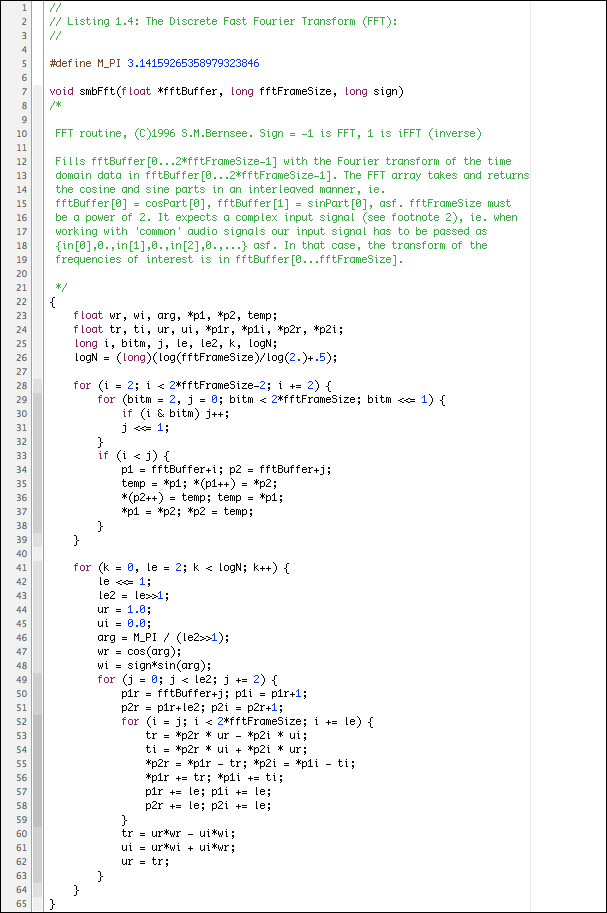*) simply speaking, trigonometric functions are functions that are used to calculate the angles in a triangle (“tri-gonos” = Greek for “three corners”) from the length of its sides, namely sinus, cosinus, tangent and the arcus tangent. The sinus and cosinus functions are the most important ones, as the tangent and arcus tangent can be obtained from sinus and cosinus relationships alone

**) Note that in the literature, due to a generalization that is made for the Fourier transform to work with another type of input signal called a ‘complex signal’ (complex in this context refers to a certain type of numbers rather than to an input signal that has a complex harmonic structure), you will encounter the sine and cosine part under the name ‘real’ (for the cosine part) and ‘imaginary’ part (for the sine part).

***) If you’re already acquainted with the DFT you may have noted that this is actually an implementation of the “real Discrete Fourier Transform”, as it uses only real numbers as input and does not deal with negative frequencies: in the real DFT positive and negative frequencies are symmetric and thus redundant. This is why we’re calculating only almost half as many bins than in the sine transform (we calculate one additional bin for the highest frequency, for symmetry reasons).

### 65 Comments to The DFT “à Pied”: Mastering The Fourier Transform in One Day

•Dave says:

Very cool. However, I don’t understand how…

frequency[bin] = (float)bin * sampleRate / (float)transformLength;

… can be meaningful.

Is something missing there?

Also, is it cool that I’m commenting on an article almost ten years after it was published?

•Bernsee says:

I’m not sure what could be your problem with the above line of code… maybe you could be more specific? What you do is you multiply the bin number by the frequency spacing in order to get the center frequency (in Hz) of the bin that you’re looking at. If your transform is of size 2048 and you’re looking at a signal that was sampled at 44.1kHz your bin frequency spacing is 44100/2048 = 21.53 Hz. The center frequency of bin #50 for instance would then be 50 * 21.53 = 1076.66 Hz.

The article is quite old but I thought it might still be useful. We have recently upgraded the web site to allow comments, so you can actually comment on all the articles no matter how old they are.

HTH, –smb

•Dave says:

I gotcha now. This is the best from zero to sixty article on DFT/FFT anywhere on the web. Thanks so much for putting it together!

•Bernsee says:

Thank you, I’m glad you like it!

•Shaurya Malhotra says:

I also saw found many other interesting articles on this site. Will read them too.
Thanks a lot..

•Mike says:

•Bernsee says:

What is it exactly that you didn’t understand? I’d be happy to clarify if you would let me know what the problem is.

•Mike says:

Well for instance, if we look at the listing 1.1, it’s not clear to me why the bins are computated that way. I dont like to just copy/paste formulas. I like to understand them, in and out.
My programming background is good, however i cannot say the same about my maths or even DSP.
Maybe the books that you have mentionned in your FAQ will help me get started?

Thanx.

•Bernsee says:

In a nutshell and without delving too deep into the maths – we know (from the article) that we need transformLength bins (sine waves) in order to fully represent the signal, this is why we loop through the bins from 0 to transformLength-1. When we’re done with that, each of the bins holds a coefficient that defines how much the input signal “looks like” a sine wave at that bin frequency (bin frequency is calculated as arg). In order to ‘compare’ the sine wave with our signal we multiply the input signal (which is stored in inputData through inputData[transformLength-1]) with a sine wave of the same length at the frequency of interest and add the results to the bin coefficient. You could see this as a filtering or correlation operation between the sine wave and our signal. Clearly, if the sine wave at that frequency looks very much like our input signal that coefficient will be large. If the signal doesn’t contain this frequency our coefficient at that bin will be zero, or very small.

Hope this helps!
–smb

•Arun Aiyer says:

When phase is calculated from PSD curve, what is the reference point for the computed phase?

•Bernsee says:

If you’re referring to the phase information that you can obtain from the transform, the reference for the bin phase is the first sample of the time domain sequence.

•Daniel P says:

I have an approximately sinusoidal signal, x, and I have calculated the abs(FFT(x)) with Matlab. My question is, what is the meaning of the magnitude I obtain? Does this magnitude have any relation to the input signal amplitude? To the number of bins? To the main frequency? Am very confused as to the meaning of FFT magnitudes and cannot find anywhere, please HELP!

•Serguei says:

A very resourceful article. We successfully built and tested a Java implementation of FFT based on this article back in 2002 in an open-source project that is still around and gets some publications out based on that. Thanks again!

•Joseph Hamilton says:

I am in the process of writing a piece of pitch detection software. I want to use FFTs to figure out the pitch of sound, but I have no idea what the byte data from a WAV means or how to relate it to this article. So my question is how does raw digital audio work? How is structured and how do I perform FFTs on it to determine the pitch?

•Bernsee says:

Well, this is a pretty broad question – answering it could easily fill a book…

In short, the bytes in a WAVE file (or any other raw PCM audio file) are a representation of the original audio signal (ie. pressure waves converted to voltage change) which has been discretely sampled in time (measured at regular intervals) and quantized to a discrete set of numbers (the measured data can vary between an established min and max value and can only take on predefined values).

How exactly the byte data is mapped to the actual signal depends on the file format, byte ordering and sample wordlength. There are several software libraries (like SoX: http://sox.sourceforge.net ) that can do most of this work for you. In most cases you will want to get a buffer of audio data in IEEE754 float format because it is very efficient for a computer to process data in this format. This is also what our example code expects.

Once you have the data from the file, you can apply your DSP processing. Note, however, that picking the largest peak from the DFT magnitudes is not a good tool for pitch detection, simply because many sounds do not have their largest peak at their fundamental frequency. So your mileage may vary if you implement a pitch detector in this way.
Hope this helps!

•Phil says:

•Doug says:

Great article and I’ve followed everything up until Step 5:

“After adding the result of the multiplication together, we will obtain the amplitude of the partial sine wave at the frequency we are looking at.”

What is the purpose of the multiplication stage? How does adding these values find the amplutude? Thanks in advance.

•magnut says:

thanksalot i now understand this thing, i got it implemented in 2.5 seconds amazing myself.

the only problem now is i dont know why i have to pass so much information into it just to get a sine wave frequency- shouldnt i just need its own cycle space exactly?

•Taj says:

@magnut: This is from the above paragraph “Conclusion”:

“The Fourier transform is independent of the signal under examination in that it requires the same number of operations no matter if the signal we are analyzing is one single sinusoid or something else more complicated. This is the reason why the Discrete Fourier transform is called a nonparametric transform, meaning that it is not directly helpful when an ‘intelligent’ analysis of a signal is needed (in the case where we are examining a signal that we know is a sinusoid, we would prefer just getting information about its phase, frequency and magnitude instead of a bunch of sine and cosine waves at some predefined frequencies).”

•Dave says:

Incredible. I have spent maybe 25 hours studying this process and this one page sum sit up in less than 45 minutes. This is amazing piece of work.

•Borges says:

thanks Bernsee for your clear tutorial
But I want to ask something.
Would you explain the logic behind the using number of bins transformLength/2
in DFT (sin and cos) instead of using transformLength.

•Taj says:

See the ***) paragraph directly above the comment area – it’s explained there. Somewhat hard to find though… 😉

•Borges says:

ok I saw that *** 🙂
but let me try to explain my problem.
for example our bins has bin frequency spacing 44100/2048 = 21.53Hz
for 2048 point DCT
but in DFT they are spaced 2*21.53 = 43.06Hz

DCT : arg = (float)bin * M_PI *(float)k / (float)transformLength; [0….transformLength ]

DFT : arg = 2.*(float)bin*M_PI*(float)k / (float)transformLength; [0….transformLength/2 ]

arg step is is changed?
Am I miss somethink?

•Taj says:

In the DFT you are using both a sine and cosine at the bin frequency, hence you can use a less dense frequency resolution. I think he has covered this on the FAQ page although I’m not sure where…

•satyam says:

hi, can anyone help me out to do Fourier Transform in Matlab please ??? am using both the simulink and programming methods but am unable to do so. anyway kind of help would be greatly apreciated, thanking u in advance…

•tb3 says:

I am following along quite well until I read the code in Listing 1.1. I understand that we are trying to find the coefficients (amplitudes) that go in front of each of our sine waves. To find these amplitudes , we are multiplying a sine wave having a known frequency (let’s say w) times each data point and then adding these products. All the coefficients are then found by cycling through this process for each one of the frequencies. To do this in the code, each coefficient is calculated by adding the product of every data point and ‘sin(arg)’. I would be fine if the arg term remained constant for each bin. However, it seems to me that the arg term (and hence the frequency of the reference sin wave) changes for each data point. Where am I getting lost?

•Taj says:

Actually, the frequency of the sinusoid at [bin] remains constant (even though arg changes because it depends on the time [k]). For each bin you’re going through all the samples of the waveform in time which is why arg changes.

•ayo says:

I must confess that after going through your lecture, I got better understanding of what the fourier series in signal processing is about. Presently, I am using matlab in a model I developed to monitor how a propagating pulse received at three different sensors can be reconstructed to get back the original pulse signal. I started by defining the pulse as a square wave which has sine and cosine functions built into it. My problem now is how to get the amplitudes and frequencies of the pulse at the sensors.

•Jeff says:

This is very helpful in explaining things in common terms! Thank you. My question is, should my input be scaled to a per unit value? So should I divide by 32768 for example with 16 bit wave audio samples? I’ve read some sources that talk about scaling the input or output, can you mention something about this?

Also, I expected that if I perform the fft on an array, and then immediately do the reverse, that I’d get the original sample values. This isn’t the case. Is this correct, or am I unclear as to how this thing should work? Thanks!

•Melissa says:

Hi,
that’s a great explanation of FT – thanks a lot!
I am currently trying to FT some data I have taken and hit upon a problem. My data shows a nice sinusoid with an amplitude decaying to zero over the time I measure it. My problem is that the peak position in the FT seems to shift slightly depending on when I stopped the measurement, i.e. depending on whether the amplitude has decayed to zero or the data is cut off before. I do not understand how cutting off the original curve should change the peak position of the FT and would be very happy about any explanations.
Thanks!

•Bill says:

Thank you very much for this tutorial (albeit 10 years later!). I wish many subjects (FIR, IIR, Zwicker loudness, etc) could be explained with the same brevity and clarity you’ve shown here. I agree withe the other posters – this is a truly great piece of work!

•Kyle Mew says:

Thanks for this – it was very clear and well explained

•Kyle Mew says:

very good – i’ve been looking for a jargon free explanation of dft and fft for ages – and this is just that – thank you

•Tato says:

Can I build a multi effects processor for my electric guitar using a DSP with the Fourier stuff and the C++ programming?

•Bernsee says:

Sure!

•Gustavo Leig says:

Thats great… what would be beautiful..at least for 1st readers would be nice to see what happens to a sin wave after the transfourm.

•Arzon says:

Fantastic tutorial, but this sentence doesn’t make any sense, to me:

“Summarizing what we have just learned, we see that the upper frequency of a sampled sine wave is every other sample being a peak and a valley and the lower frequency bound is half a period of the sine wave which is just fitting in the number of samples we are looking at.”

•Gustav says:

Thanks a lot for taking the time to dumb this down and make it “programmatic”. 😀

•Chris Smith says:

Thank you for this. Fantastic article.

Having encountered FFT’s in the past and never truly understanding them (thanks to some poor tuition), this hits the spot. If only university text books and Knuth were as concise as this 🙂

•Gabriel says:

Wonderful tutorial, I read before another excellent tutorial for Fourier transforms by Robi Polikar, but it deals more with Wavelets (a better way than Short Time Fourier Transforms).

The only place where I’ve been confused on this one is the computation of Magnitude and the Phase in the code. Where does it come from?

Thanks again for the tutorial.

•Said says:

It’s a great tutorial about the DFT, however I cannot see any details about the phase correction (compensation) when DFT is dealing with sine wave signal, as it introduce a phase shift to the signal. I read many books where they said you have to compensate for 90 degree phase shift and i dont thing it is a general way to do it.

•Bernsee says:

I don’t think that I understand your question. The DFT per se does not introduce a phase shift into the signal – if you iDFT a transformed block of data you will get back your original signal (a very good approximation of it, that is).

•Jai says:

It’s probably not necessary since there are so many kudos here, but I just wanted to add a heart thanks for a fantastic “executive summary”. I am sure that was a lot of work to condense. Not only is this a Fourier Transform for Dummies, it’s also a great Fourier Transform for Dummies with ADHD. 🙂

•Christian Brumm says:

This is a VERY good and gentile introduction to the Fourier Transform. The sample code helps a lot and it is a good exercise to derive the math from it. Please keep this article up, I think it will still be useful to many people in the years to come!

•Ed says:

Very nice tutorial.

However I don’t quite understand why “we see that the upper frequency of a sampled sine wave is every other sample being a peak and a valley and the lower frequency bound is half a period of the sine wave which is just fitting in the number of samples we are looking at.”

Isn’t it possible that a partial sinusoid can have a very high frequency that there can be several periods between two sampling points?

•Bernsee says:

Yes, this is indeed possible. However, such a signal cannot be correctly described by our digital representation. If you have several signal periods between adjacent sampling points then you will not be able to reconstruct that signal and aliasing will occur. Aliasing is an effect that causes frequencies that are higher than the highest frequency that can be represented to appear as low frequencies. The following picture should make this clear:The black waveform has multiple periods between the sampling points (blue lines). So if we sample that waveform (= measure it at the blue intervals) it will effectively look like a different waveform that has a much lower frequency (red dotted waveform).

In essence what this means is that once you get to play with your sampled signal all frequencies that are higher than the highest possible frequency (the one with adjacent peaks and valleys located on the sample grid) have either been removed, or appear as lower frequencies due to aliasing.

•Loki Clock says:

Note, this half-period resolution limit is what’s known as the Nyquist or cut-off frequency. This is a reflection of quantization itsself, which need not be digital. If the universe had a minimum unit of mass, but not a maximum frequency, you could potentially create aliasing by letting a wave with a wavelength twice or less than the length of that minimum unit propagate through it. I have no idea what that would do, or if there are any problems with that sentiment, but it’s still crazy to think about!

To the author, I am looking forward to reading this over break. It shall surely be a treat, as I’ve been needing an explanation of a Fourier transform for the very purpose of programming one in C++.

•Paul says:

The Nyquist limit is a result of sampling, not quantization. Not sure what you’re getting at with the minimum mass/maximum frequency limit of the universe but I think these limits have not been established yet…

-P

•Aran says:

im sure these limits have been established, the universe does exist…

•Paul says:

Yes, but I’m not sure if scientists have been able to measure them yet… they are still in the process of repairing the LHC.

-P

•yose says:

This is an excellent article. Finally, I can get a clear sense of what is happening and what a fourier transform involves of. Was being confused with the convoluted ways most other sources try to explain things (by going to deep into the math-sides of things).

•Jack Kinsella says:

Fantastically well written.

By the way the internet already recognizes this article’s merit – it’s one of the most popular articles tagged “math” on delicious.

Please do more tutorials in future.

•Bernsee says:

Thank you, I appreciate your feedback! I am working on more tutorials at the moment – if you have a specific topic that you would like to read about please let me know (either by emailing or posting here).

•Kris Bishop says:

I have preiously studied this at University, passed the exam, but never understood it until I read your explanation.

very well done

Thanks

•Mark says:

Awesome! This has helped me a lot. Thank you!

•shabtronic says:

great web page – starting to understand the fft now!!

what’s in Bin 0 – is that a DC offset or overall magnitude?

•Bernsee says:

Thank you for your feedback. In our example listing 1.2 bin #0 contains only the DC component.

Some FFT implementations put both DC and fs/2 into bin #0 (as real and imaginary part) because both are real-valued and that way you can use N/2 complex transform bins instead of N/2+1.

•akhil Gada says:

ur totarial is verry good thnx a lot for that.
wat is the significance of DC component of freq domain signal ? Where is it used in context of sound processing?
“normalize the spectrum, treat it as a
probability density function, and finally obtain the spectral
entropy, Hf , by,
Hf = ? summation over i( pi log pi ) where i =1 to n. It appears in one of the research paper related to audio data processing .We are implementing project on activity recognition using microphone on Android .Kindly send me your response on my email : agada@usc.edu or gadaakhil@gmail.com
Thnx a lot
Akhil
Univ of southern calif LA ,USA

•alfi pacho says:

I know what is DFT, but what is DFT “à Pied”: ?

•Paul says:

It’s French and means that you learn the DFT exploring it “by foot”.

•kraymer says:

maybe the author meant “pas à pas” ?? (one step at a time)

•Paul says:

It’s “by foot”, = “à pied”

•maht says:

oOOO thank you.

Although I knew what an FFT was and how it works I’ve never come across listing 1.3 before, it’s always on to Cooley Turkey and I’d never quite worked out how to get to freq[] maq[] phase[] from the butterfly.

•Nate says:

I am just a little fuzzy on this. When a fft is done on a signal why are there negative numbers of frequencies occurring on the y-axis? Is this just representing the amplitude of the negative sinusoid waves used?

•Jose says:

Outstanding post! Very good explanation, it is very useful. Thanks a lot 🙂